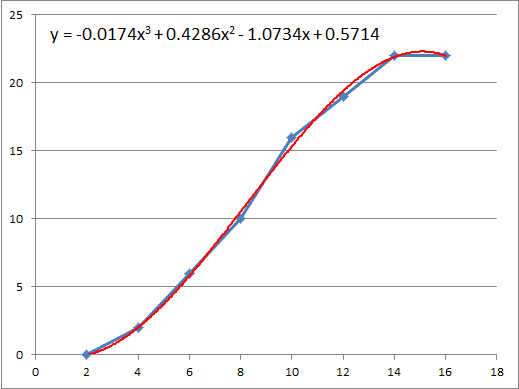# Instantaneous Acceleration from a Velocity-Time graph

## Homework Statement

A test driver is testing a new model car with a speedometer calibrated to read m/s rather than mi/h. The following series of speedometer readings were obtained during a test run along a long, straight road:

Time (s): 0 2 4 6 8 10 12 14 16
Speed (m/s): 0 0 2 6 10 16 19 22 22

I.e.,
(0 s, 0 m/s)
(2, 0)
(4, 2)
(6, 6)
(8, 10)
(10, 16)
(12, 19)
(14, 22)
(16, 22)

(a) Compute the average acceleration during each 2-s interval. Is the acceleration constant? Is it constant during any part of the test run?
(b) Make a velocityx-time graph of the data above, using scales of 1 cm = 1 s horizontally and 1 cm = 2 m/s vertically. Draw a smooth curve through the plotted points. By measuring the slope of your curve, find the instantaneous acceleration at t = 9 s, 13 s, and 15 s.

## Homework Equations

Average Acceleration
a = (Δvx)/Δt

Instantaneous Acceleration
a = dvx/dt

## The Attempt at a Solution

I know how to get the answers for (a) and these are 0m/s/s, 1 m/s/s, 2 m/s/s, 2 m/s/s, 3 m/s/s, 1.5 m/s/s, 1.5 m/s/s, and 0 m/s/s. Acceleration is constant at 2 m/s/s and 1.5 m/s/s.

For (b), I made a graph as specified and tried finding the slopes of the tangent lines at t = 9 s, 13 s, and 15 s. My answers have not matched the book's, which are 2.5 m/s/s, 1.5 m/s/s, and 0 m/s/s, respectively. It makes sense that the instantaneous acceleration at 15 s is 0 m/s/s as there is no rise in the tangent line at t = 15 s and so the slope equals 0.

Thanks in advance.

## Answers and Replies

BvU
Science Advisor
Homework Helper
Hello Aff, and (a belated) welcome to PFYour answers don't match the book, you say. What are your answers ?

I looked at the plot and I can imagine that smoothing would move the point (10, 16) a bit down, e.g. to (10,15). Then the slope would match the 2.5 m/s2.

For the other two I wouldn't expeect your answers to deviate very much. am I right ?

Chestermiller
Mentor
Your calculated accelerations are correct, and match the book answers at 13 and 15 s. The book answer is wrong at 9 s, but yours is correct there too.

Chet

Hey BvU,

Thanks for the welcome!

My answers varied as I tried quite a few different tangent lines for each point. I was able to get an instantaneous accelerations of 3.5 m/s/s and ~3.0 at 9 s, 1.5 m/s/s and ~1.33 m/s/s at 13 s, and of course 0 m/s/s at 15 s.

@Chestermiller

Is finding the slope of the tangent lines at each point a correct way of finding the instantaneous acceleration at those points?

Chestermiller
Mentor
Hey BvU,

Thanks for the welcome!

My answers varied as I tried quite a few different tangent lines for each point. I was able to get an instantaneous accelerations of 3.5 m/s/s and ~3.0 at 9 s, 1.5 m/s/s and ~1.33 m/s/s at 13 s, and of course 0 m/s/s at 15 s.

@Chestermiller

Is finding the slope of the tangent lines at each point a correct way of finding the instantaneous acceleration at those points?
It's a very accurate approximation for the center of each time interval. With discrete data like this, it's the best you can do.

BvU
Science Advisor
Homework Helper
Hi folks,

This is pretty pedantic, but I liked this exercise, so there. Some remarks:

The story is about an accelerating car, so gear shifts can be a reason for a not-all-that-smooth acceleration versus time.
That aside, the exercise tekst wants a smooth curve drawn. I'm no artist, so I let a program (Excel) do the work: a third order polynomial does a reasonable job. Again, it doesn't know the driver takes his foot off the pedal at 14 s, so it overshoots, but never mind. And the four-digits are way too much accuracy (And yes, I did find the button to reduce that, but too late). See the red line.I am pleased to see it indeed draws the line through (10, 15) -- but, again, it doesn't know about possible gear shifts.
Slope of line = derivative = instantaneous acceleration at t = 9, 13, 15s: 2.4, 1.25 and 0.04, respectively.
In view of the accuracy of the data, 2.5, 1.5 and 0 are adequate.

3.5 or 3 m/s2 at t = 9s to me seems on the high side. Unless it's a pretty bad driver, fumbling that gear shift -- but in that case a smooth line isn't justified.

Chestermiller
Mentor
Ah. I didn't notice the part about putting a smooth curve through the data.

Chet